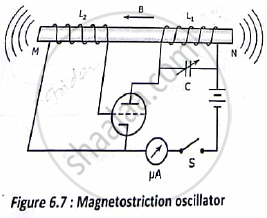BE Civil Engineering Semester 1 (FE First Year)University of Mumbai
Share
Notifications

View all notifications
Books Shortlist
Your shortlist is empty

# Explain the Principle, Construction and Working of a Magnetostriction Oscillator. - BE Civil Engineering Semester 1 (FE First Year) - Applied Physics 1

Login
Create free account

Forgot password?
ConceptMagnetostriction Oscillator

#### Question

Explain the principle, construction and working of a Magnetostriction Oscillator.

#### Solution

This is based on the principle of magnetostriction effect.

⦁ A ferromagnetic rod MN is clamped in the middle. Two coils L1 and L2 are wound round its two halves.

⦁ The coil L is connected in the plate – cathode circuit and the coil L2 is connected in the grid cathode circuit of a triode valve oscillator.
⦁ The capacitor C has a variable capacitance .
⦁ As the circuit is switched on the plate current Ip starts flowing through coil L1 producing  magnetic field B along the axis of the rod as shown:-

⦁ Now the rod is placed in a magnetic field along its length as a result of which its overall length will change by a small amount.

⦁ As the length changes the flux linked with Land L2 also changes giving rise to an induced emf , e= -dφ/dt across L2 .

⦁ The induced emf is fed to the grid-cathode circuit and the oscillator valve gives an amplified alternating voltages as output to the plate-cathode circuit causing an alternating plate current.

⦁ Due to the alternating current flowing through coil L1 the magnetic field becomes an alternating magnetic field giving rise to the vibration of the rod.
⦁ The frequency of mechanical vibration of the rod is given by

f_(rod) = p/(2L) √Y/ρ

Where p = mode of vibration

L = length of the rod.
rod

Y = Young’s modulus of the material of the rod and, density of the material of the rod.For first harmonic p =1 and the frequency of vibration becomes

f(rod) = p/(2L) √Y/ρ

The frequency of the triode valve oscillator is given by
f_(osc) = 1/( 2π√L_1 C)

By varying the capacitance C of the variable capacitor the oscillator frequency , fosc can be  adjusted to the mechanical frequency of the rod frod . In the case

frod  =  fosc
And resonance takes place.

⦁ At resonance the rod vibrates vigorously emitting ultrasonic waves from both its ends.
⦁ By adjusting the length of the rod and the capacitance of the variable capacitor ultrasonic waves of frequency up to 300KHz can be produced .

Is there an error in this question or solution?

#### APPEARS IN

Solution Explain the Principle, Construction and Working of a Magnetostriction Oscillator. Concept: Magnetostriction Oscillator.
S